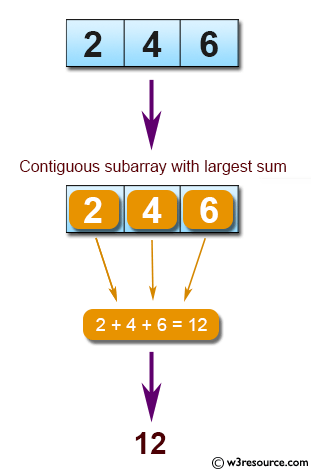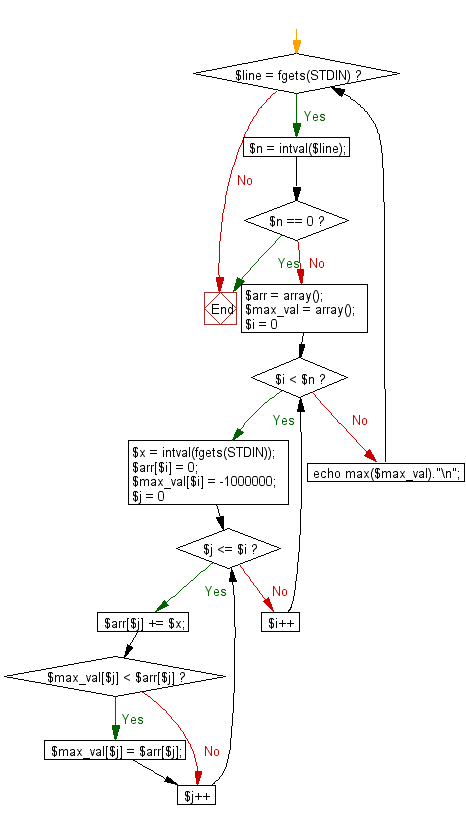﻿ PHP Exercise: Find the maximum sum of a contiguous subsequence from a given sequence of numbers a1, a2, a3, ... an - w3resource

# PHP Exercises: Find the maximum sum of a contiguous subsequence from a given sequence of numbers a1, a2, a3, ... an

## PHP: Exercise-57 with Solution

Write a PHP program to find the maximum sum of a contiguous subsequence from a given sequence of numbers a1, a2, a3, ... an. A subsequence of one element is also a continuous subsequence.

Input:You can assume that 1 ≤ n ≤ 5000 and -100000 ≤ ai ≤ 100000.
Input numbers are separated by a space.
Input 0 to exit.

Pictorial Presentation:Sample Solution: -

PHP Code:

``````<?php
while(\$line = fgets(STDIN)){
\$n = intval(\$line);
if(\$n == 0){
break;
}
\$arr = array();
\$max_val = array();
for(\$i = 0; \$i < \$n; \$i++){
\$x = intval(fgets(STDIN));
\$arr[\$i] = 0;
\$max_val[\$i] = -1000000;
for(\$j = 0; \$j <= \$i; \$j++){
\$arr[\$j] += \$x;
if(\$max_val[\$j] < \$arr[\$j]){
\$max_val[\$j] = \$arr[\$j];
}
}
}

echo max(\$max_val)."\n";
}
?>
```
```

Sample Input:
6
-4
-2
5
3
8

Sample Output:

```16
```

Flowchart:PHP Code Editor:

Have another way to solve this solution? Contribute your code (and comments) through Disqus.

What is the difficulty level of this exercise?

Test your Programming skills with w3resource's quiz.

﻿

## PHP: Tips of the Day

Mutates the original array to filter out the values specified

Example:

```<?php
function tips_pull(&\$items, ...\$params)
{
\$items = array_values(array_diff(\$items, \$params));
return \$items;
}

\$items = ['x', 'y', 'z', 'x', 'y', 'z'];
print_r(tips_pull(\$items, 'y', 'z'));
?>
```

Output:

```Array
(
 => x
 => x
)
```